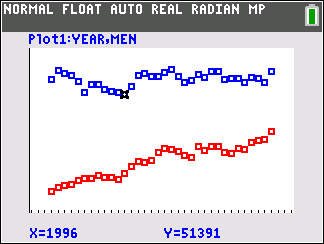# Activities

•• ##### Subject Area

• Math: Mathematical Modeling: Prediction

6-8
9-12

50 Minutes

• ##### Device
•TI-84 Plus C Silver Edition
•TI-84 Plus CE

## Modeling Median Incomes#### Activity Overview

In this TI-84 family activity, students investigate and examine the data underlying the claim that women’s income is catching up to men’s income.

#### Objectives

Students will be able to:

• Model a contextual situation mathematically and use the model to make a prediction
• Represent two quantitative variables on a scatter plot, and describe how the variables are related.
• Find and interpret linear equations to model relationships between two quantitative variables;
• Use proportional relationships to solve real-world and mathematical problems